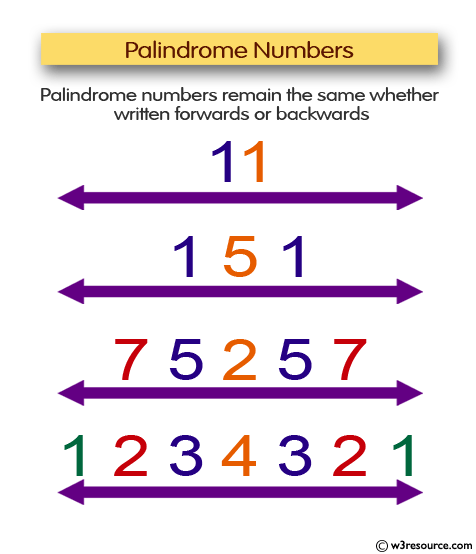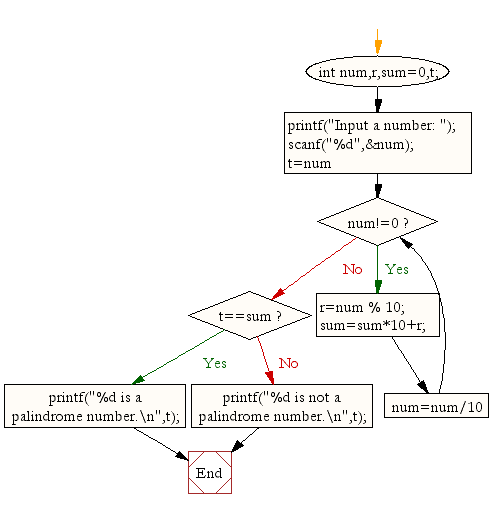﻿ C Program: Check whether a number is a palindrome or not - w3resource# C Exercises: Check whether a number is a palindrome or not

## C For Loop: Exercise-38 with Solution

Write a program in C to check whether a number is a palindrome or not.

Pictorial Presentation:Sample Solution:

C Code:

``````#include <stdio.h>

void main(){
int num,r,sum=0,t;

printf("Input a number: ");
scanf("%d",&num);

for(t=num;num!=0;num=num/10){
r=num % 10;
sum=sum*10+r;
}
if(t==sum)
printf("%d is a palindrome number.\n",t);
else
printf("%d is not a palindrome number.\n",t);

}
```
```

Sample Output:

```Input a number: 121
121 is a palindrome number.
```

Flowchart:C Programming Code Editor:

Improve this sample solution and post your code through Disqus.

What is the difficulty level of this exercise?

Test your Programming skills with w3resource's quiz.

﻿